Pandas Fillna Examples: Filling in Missing Data

# Pandas Fillna Examples: Filling in Missing Data

Last updated:

## Fill with value

This is the simplest way to use fillna, just call df.fillna(<value-to-fill>)

Example: Fill with 0:

import pandas as pd
import numpy as np

df = pd.DataFrame({
'col1': [1.0,   2.0,  3.0,   np.nan, None ],
'col2': [1,     2,    3,     4,      5    ],
'col3': ['foo', None, 'baz', 'quux', 'bax']
})

df.fillna(0)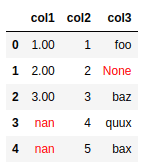BEFORE: dataframe has null-ish values
like np.nan and None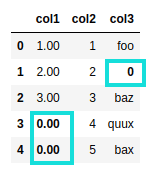AFTER: null-ish values replaced by zero but note
that column types are unchanged

## Specific column

Pass a dict of the form {col_name: value_to_fill} to fillna():

Example: Fill nulls with "-" in col1 only:

import pandas as pd
import numpy as np

df = pd.DataFrame({
'col1': [1.0,   2.0,  3.0,   np.nan, None ],
'col2': [1,     2,    3,     4,      5    ],
'col3': ['foo', None, 'baz', 'quux', 'bax']
})

df.fillna({"col1": "-"})BEFORE: dataframe has null-ish values
like np.nan and None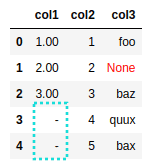AFTER: only col1 has had its null-like
values replaced by "-". Other columns are unchanged

## Specific row

Use df.loc[<row_index>, df.columns] and map instead:

Example: Fill all null-like cells in row 1 with "--"

import pandas as pd
import numpy as np

df = pd.DataFrame({
'col1': [1.0,   np.nan, 3.0,   np.nan, None ],
'col2': [1,     2,      3,     4,      5    ],
'col3': ['foo', None,  'baz', 'quux', 'bax']
})

index_to_fill = 1
value_to_fill = "--"

df.loc[index_to_fill,df.columns] = df.loc[index_to_fill,:].map(lambda value: value_to_fill if pd.isna(value) else value)
df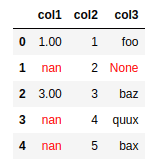BEFORE: original dataframe with several null-like values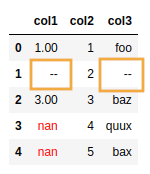AFTER: only values in the row indexed by 1
were filled with "--"
(other rows left unchanged)

## Multiple columns

To fill nulls in multiple specific columns, pass a dict to fillna

import pandas as pd
import numpy as np

df = pd.DataFrame({
'col1': [1.0,   2.0,  3.0,   np.nan, None ],
'col2': [1,     2,    3,     4,      5    ],
'col3': ['foo', None, 'baz', 'quux', 'bax'],
'col4': ['xxx', None, None,  None,   'xxx'],
})

df.fillna({"col1": 0, "col3": "--"})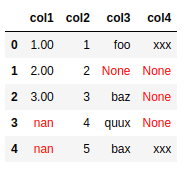BEFORE: several columns have null-like values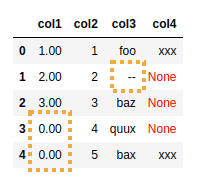AFTER: only col1 and col3 have had
their nulls filled with 0 and "--",
respectively

## Fill inplace

Just pass inplace=True to make fillna() return None instead of the modified dataframe:

import pandas as pd
import numpy as np

df = pd.DataFrame({
'col1': [1.0,   2.0,  3.0,   np.nan, None ],
'col2': [1,     2,    3,     4,      5    ],
'col3': ['foo', None, 'baz', 'quux', 'bax']
})

# returns nothing
df.fillna(0, inplace=True)


## Fill with another column

This is sometimes called coalesce in other tools such as Apache Spark.

Use a dict and pass the column you want as the values:

Example: Fill nulls in col1 using values in col2

import pandas as pd
import numpy as np

df = pd.DataFrame({
'col1': [1.0,   2.0,  3.0,   np.nan, None ],
'col2': [1,     2,    3,     4,      5    ],
'col3': ['foo', None, 'baz', 'quux', 'bax'],
})

df.fillna({'col1': df['col2']})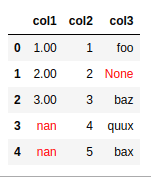BEFORE: original dataframe with some null values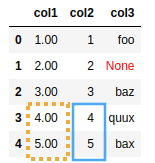AFTER: every missing value in col1 was filled with the
equivalent value in col2
This is sometimes referred to as coalescing in
tools such as Apache Spark

## Fill with another dataframe

If you have another dataframe to serve as the replacement values, you can use that by calling df.fillna(another_df):

Example: Fill null-like values in df with the matching values in replacement_df

import pandas as pd
import numpy as np

df = pd.DataFrame({
'col1': [1.0,   2.0,  3.0,   np.nan, None ],
'col2': ['foo', None, 'baz', 'quux', 'bax'],
})

replacement_df = pd.DataFrame({
'col1': [0.0,  0.0,  0.0,  0.0,  0.0 ],
'col2': ['--', '--', '--', '--', '--'],
})

df.fillna(replacement_df)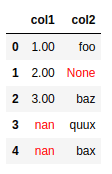df is the base dataframe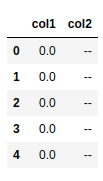replacement_df is the dataframe with the values to be used
(note that the column names and the index
match the ones in the base dataframe!)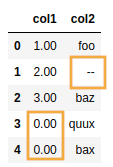AFTER: null-like values in df have been replaced with the
matching values in replacement_df

## Fill values matching condtion

By default, fillna will fill null-like values, which inlude np.nan, None, etc.

To fill or replace other values, use df.where(<func>, <value_to_replace>) instead:

Example: replace all odd numbers with a string "ODD":

import pandas as pd
import numpy as np

df = pd.DataFrame({
'col1': [1,  2,  3,  4],
'col2': [11, 12, 13, 14],
})

# note that the function must evaluate to FALSE
# to trigger the replacement
df.where(lambda value: value %2 == 0, "ODD")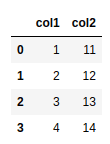BEFORE: even and odd numbers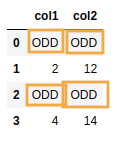AFTER: use where to pass a function that returns a boolean
value signalling which values should be replaced
by the provided value

## Null-like values

These are all values the fillna function considers to be "null-like":

Value
pandas.NaT
pandas.NA
numpy.nan
None

## Fill other values

One way to do this is to use df.replace to turn the values you wish to consider as null-like into np.nan or something like that and then use fillna:

Example: consider the string "-" as null-like and use fillna

import pandas as pd
import numpy as np

df = pd.DataFrame({
'col1': [np.nan, "-",   3.0,  np.nan, None ],
'col2': [np.nan, "foo", 'baz', pd.NaT, "-"],
})

df = df.replace({"-":np.nan})

df.fillna("XXX")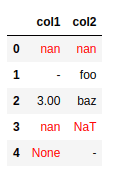BEFORE: in addition to normal null-like values
like np.nan and None, we would
also like to consider "-" as a null-like value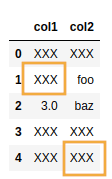AFTER: both the normal null-like values and the
cells that had "-" had their values
replaced with "XXX"